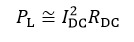# DC Wiring

This routine computes the power lost due to Joule heating losses within the DC portion of the array. The resulting current and voltage is that seen at the inverter terminals. The resistance can also be estimated if a percent Joule loss (PJL) at STC is provided instead of the equivalent bulk resistance. The resistance from a percent Joule loss is estimated as follows from the module’s rated STC current and voltage and number of series & parallel connections in the DC Field: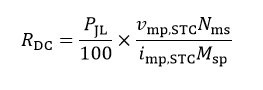## Inputs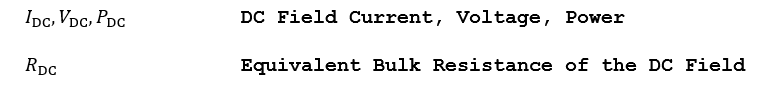## Outputs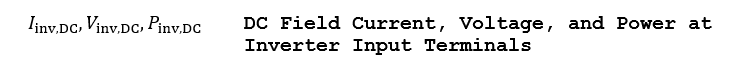## Algorithm (Delta Series Resistance)

1.) Given an equivalent bulk resistance RDC, find the delta series resistance seen by one module as follows: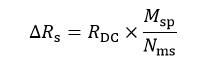2.) This ΔRS is then added to the series resistance in the one-diode model.

3.) An approximation of the power dissipated by the bulk resistance can be obtained from the DC Field power seen by the inverter is as follows: